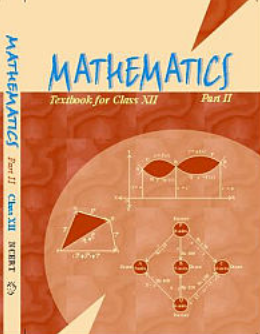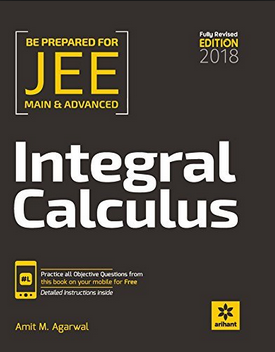# Integral Calculus (Weightage 9%)   Share

### Topics from Integral Calculus

• Integral as limit of a sum, fundamental theorem of calculus (4 concepts)
• Determining areas of the regions bounded by simple curves in standard form (9 concepts)
• Properties of definite integrals, evaluation of definite integrals (21 concepts)
• Integral as an anti-derivative, fundamental integrals algebraic, trigonometric, exponential and logarithmic functions (37 concepts)
• Integration by substituion using trigonometric identities (14 concepts)
• Indefinite Integral (22 concepts)
• Definite Integral (11 concepts)
• Area (4 concepts)

### Important Books for Integral Calculus

••Exams
Articles
Questions